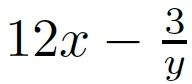# Sample Midterm

These are sample questions that are very similar to the ones I will ask on the midterm. The midterm will be (slightly) longer.

1. Which of the following Python identifiers are legal variable names? For those that are not, say why not.
1. x
2. Celsius
3. spam3
4. <hello>
5. question?
2. What is the data type (int, float, string, boolean) of the following? Please evaluate them.
1. 3.9 + 6
2. 5 + 1 > 7 or 2 == 1 + 1
3. int("3")
4. 7 / 2
5. 8 // 3
3. Write the following formulas in Python.
1. a2
2.3.4. What does the following program print?
```for i in range(1, 5):
x = (-1) ** i * i
print(i, x)
```
5. Convert the following into a “for” loop.
```i = 1
while i <= 10:
print(i)
i += 3
```
6. When I ran the following program:
```x = int(input("Enter a number: "))
y = 1 / x
print("The reciprocal of", x, "is", y)
```
I got the following error message:
```Traceback (most recent call last):
File "x.py", line 2, in
y = 1 / x
ZeroDivisionError: int division or modulo by zero
```
What was the specific error? Where did it cause the exception? And what did the user type?

 You can also obtain a PDF version of this. Version of April 21, 2014 at 12:30PM